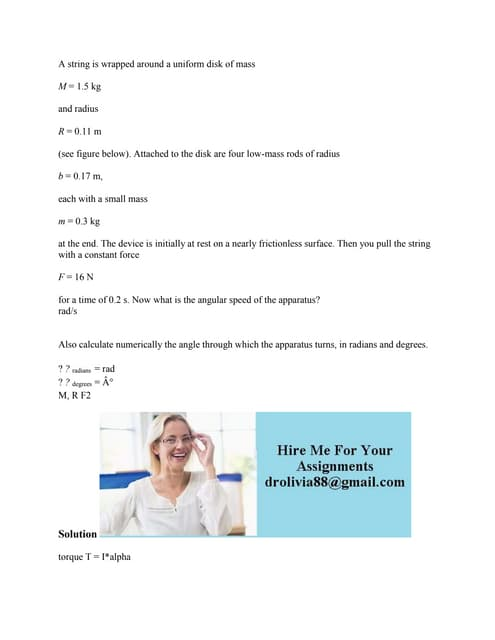Anúncio

# A stationary tennis ball is dropped from a height of 1 m- As a result-.docx

3 de Feb de 2023Próximos SlideSharesA string is wrapped around a uniform disk of mass M - 1-5 kg and radiu.docx
Carregando em ... 3
1 de 1
Anúncio

### A stationary tennis ball is dropped from a height of 1 m- As a result-.docx

1. A stationary tennis ball is dropped from a height of 1 m. As a result, the ballâ€™s inertia will remain constant but its momentum will change. True or false? A stationary tennis ball is dropped from a height of 1 m. As a result, the ballâ€™s inertia will remain constant but its momentum will change. True or false? A stationary tennis ball is dropped from a height of 1 m. As a result, the ballâ€™s inertia will remain constant but its momentum will change. True or false? Solution It is true that the ballâ€™s inertia will remain constant but its momentum will change, As the ball is initially at the height of h = 1 m, so the ball will have gravitational potential energy associated to it as PE = mgh, Now, when we will drop the ball from height h, the potential energy of the ball will convert into the kinetic energy (as accordance to law of conservation of energy) as KE = 1/2 * mv 2 , hence the velocity of the ball change and in our case it will increase from v = 0 m/s to V max at the ground, and so there will be change of momentum of the ball due to change in the velocity although mass of the ball will remain constant.
Anúncio